Liu, Y., Zhou, J., Fu, W., Zhang, B., Chang, F., and Jiang, P. (2020). "The effect of bamboo surface roughness of cutting parameters on the bamboo milling," BioRes. 15(4), 8323-8338.

#### Abstract

The surface roughness of bamboo is a factor that determines the processing quality. The effects of spindle speed, feed speed, and cutting depth on the surface roughness of bamboo were studied using the orthogonal design test method. The results were analyzed using range analysis, main effect analysis, interaction analysis, and variance analysis to determine the effect of cutting parameters on the surface roughness of bamboo. The feed speed had a greater effect on the surface roughness of the bamboo in the tangential section; the spindle speed had a greater impact on the surface roughness of the bamboo in the cross section. The cutting depth had a greater impact on the surface roughness of the bamboo in the radial section. For the surface roughness on the tangential, cross, and radial sections of the bamboo, there was a relationship with the cutting parameters. A mathematical model for the analysis of surface roughness was established by response surface method. Also, contour and surface plots based on regression models showed the correlation between surface roughness and all possible pairwise combinations of three cutting parameter variables. The cutting technology with the best surface quality was determined by optimization analysis.

The Effect of Bamboo Surface Roughness of Cutting Parameters on the Bamboo Milling

Yanhe Liu,a,b Jianbo Zhou,a,b,* Wansi Fu,Bin Zhang,a,b Feihu Chang,a,b and Pengfei Jiang a,b

The surface roughness of bamboo is a factor that determines the processing quality. The effects of spindle speed, feed speed, and cutting depth on the surface roughness of bamboo were studied using the orthogonal design test method. The results were analyzed using range analysis, main effect analysis, interaction analysis, and variance analysis to determine the effect of cutting parameters on the surface roughness of bamboo. The feed speed had a greater effect on the surface roughness of the bamboo in the tangential section; the spindle speed had a greater impact on the surface roughness of the bamboo in the cross section. The cutting depth had a greater impact on the surface roughness of the bamboo in the radial section. For the surface roughness on the tangential, cross, and radial sections of the bamboo, there was a relationship with the cutting parameters. A mathematical model for the analysis of surface roughness was established by response surface method. Also, contour and surface plots based on regression models showed the correlation between surface roughness and all possible pairwise combinations of three cutting parameter variables. The cutting technology with the best surface quality was determined by optimization analysis.

Keywords: Bamboo; Orthogonal design; Spindle speed; Feed speed; Cutting depth; Mathematical model; Surface roughness

Contact information: a: Institute of New Forest Technology, Chinese Academy of Forestry, Beijing, China, 100091; b: Beijing Forestry Machinery Research Institute of State Forestry and Grassland Administration, Beijing, China, 100029; *Corresponding author: zhoujianbol@126.com

INTRODUCTION

As a renewable material with excellent properties, bamboo has high strength, hardness, stiffness, and a short production cycle. It is widely used in construction, furniture, food and other fields. It is a kind of “green” raw material that can replace wood.

Bamboo milling refers to the surface preparation procedure that occurs during the preliminary processing stage of bamboo. The preparation of the bamboo surface is done to achieve good processing surface quality, and as a bamboo milling performance evaluation standard, the product surface roughness is usually considered. Surface roughness has a significant effect on the processing quality and the aesthetics of bamboo products. Xiao et al. (2016) studied the effect of three cutting parameters of spindle speed, feed speed, and cutting depth on the surface roughness of AISI 1045 steel in the hard turning test of YT5 tool using the orthogonal experimental method, established are lative regression model and also optimized for the best cutting conditions. Sarıkaya and Güllü (2014) designed L16 (43 × 21) experiments based on Taguchi principles. The effect of different cutting parameters on the surface roughness of AISI 1050 steel during turning were studied. The experiments were carried out under the conditions of dry cutting (DC), conventional wet cooling, and MQL, respectively. The mathematical model of surface roughness was established and optimized using the response surface method. Çelik et al. (2016) used CVD and PVD coated carbide tools to turn Ti-6Al-4V alloy, and they studied the effect of different spindle speed, feed speed, cutting length and cutting depth on tool wear and surface roughness. Saini et al. (2012) studied the effect of cooling conditions, spindle speed, feed speed and cutting depth on tool wear and surface roughness when turning AISI H11 tool steel with ceramic tools. The response surface method was used to model and predict the surface roughness and tool wear at different cutting conditions.

Hanafi et al. (2012) employed the gray correlation theory and Taguchi optimization in tin tool cutting peek-cf30 to obtain the cutting parameter combination that achieved the lowest power and the best surface roughness while maintaining the machining quality of the workpiece and decreasing pollution of the environment at the same time. Yan and Li (2013) used weighted gray correlation analysis and response surface method to study the multi-objective optimization of the cutting parameters between energy, production efficiency, and cutting quality. They obtained the optimal surface roughness, material removal rate, and cutting energy consumption. They concluded that the traditional objective optimization method cannot meet the requirements of sustainable development of machining. Debnath et al. (2016) used Taguchi orthogonal test method to design a test scheme. TiCN + Al2O3 + TiN coated carbide tool was used to turn low carbon steel. The effects of the different cutting parameters and cutting fluid parameters on the surface roughness and tool wear were studied.

Artificial neural network is an algorithmic mathematical model which imitates the behavior characteristics of animal neural network and carries out distributed parallel information processing. It can better design experimental scheme and process experimental data. Tiryaki et al. (2016) investigated using artificial neural network to derive a mathematical model that is based on the data of the various processing parameters on the surface roughness of wood in abrasive machining process; it was found that the impact of power consumption determined the optimal cutting parameter value to minimize cost. Stanojevic et al. (2017) studied the influence of the feed speed, cutting depth, and front angle on surface roughness and power consumption by using the neural fuzzy method and represented it in a model. The results of this study can be applied in the wood industry to minimize energy consumption and experimental costs. Goli et al. (2018) proposed a novel experimental method to evaluate the specific cutting coefficient in the isotropic and orthotropic milling of wood materials and also to optimize and compare materials and processes.

Li et al. (2019) studied the cutting performance of micro-grain in wood cutting, the cutting force of turning, and how the surface friction coefficient between the front cutting surface and chip can be reduced. The energy consumption was reduced and the processing quality was improved. Kamboj et al. (2020) studied the effect of thermal modification on the surface quality and the cutting capability of eucalyptus wildebeest processing machines, and carried out edge milling at different cutting speeds, front angles, and feed speeds, to optimize and analyze the best surface quality and the amount of energy consumed. Using the single factor analysis method, Guo (2009) studied the effect of cutting tool rake angle, milling speed, milling depth, radial surface, and chord surface of longitudinal cutting on the milling performance of bamboo. Wu (2006) studied the effect of bamboo’s physical properties and cutting parameters on the cutting quality using a high-speed camera and micro measurement technology, which achieved the purpose of reducing cost and improving quality.

At present, a large number of researchers are engaged in metal cutting and wood cutting research, and few are engaged in bamboo cutting research, but the research on bamboo cutting is also very important. In this paper, the effect of cutting parameters (spindle speed, feed speed, and cutting depth) on the surface roughness of bamboo was studied using an orthogonal experiment. The mathematical model of the relationship between cutting parameters and the surface roughness of bamboo was established, and the cutting technology of the surface roughness of bamboo was optimized. Furthermore, the spindle speed, feed speed and cutting depth corresponding to the best cutting technology under the experimental conditions are determined.

EXPERIMENTAL

Selection of Cutting Parameters and Their Levels

This study was conducted in the SMART five-axis machining center established by Paolino Bacci in Italy. The experimental material was bamboo plywood, which is formed by a bamboo cross and side hot pressing, as shown in Fig. 1. The bamboo cross and side hot pressing test material length × width × height were 15 cm × 15 cm × 2 cm. The workpiece of bamboo plywood was milled with a manganese plated carbide cutter. The view of the cut area layout is shown in Fig. 2. The bamboo material was milled with a 12T thin face milling cutter with 27 flat knives produced by Hangzhou Xiaoshan Baolifeng bamboo cutting tool factory (Hangzhou, China). The effect of three cutting parameters—spindle speed, feed speed, and cutting depth—on the surface roughness of bamboo was considered. The cutting parameters and their levels are shown in Table 1.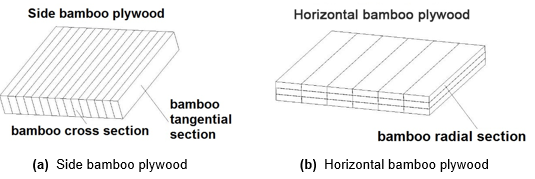Fig. 1. Bamboo plywood work piece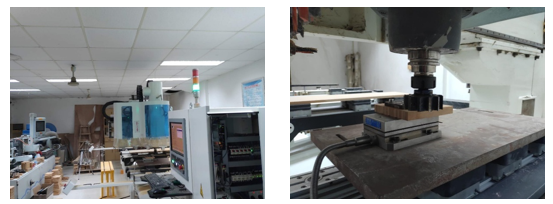Fig. 2. SMART five-axis machining center

The contact-type measuring instrument S-NEX001SD-12 (Olympus, Co. Ltd., Japan) was used to measure the surface roughness and measurement at three different locations reduces the error. The average values of Ra (arithmetic average of absolute values of roughness profile) were considered for analysis. Ra was used to measure the variables: the strings, the end face, and the diameter of the bamboo workpiece. An average value of the surface roughness was calculated in five sets each for the tangential section surface roughness of the bamboo, Ra1, the cross-section surface roughness of the bamboo, Ra2, and the radial surface roughness, Ra3. The cut-off length and sampling number of surface roughness measurement are 1 mm and 5 times respectively.

Table 1. Processing Parameters and Levels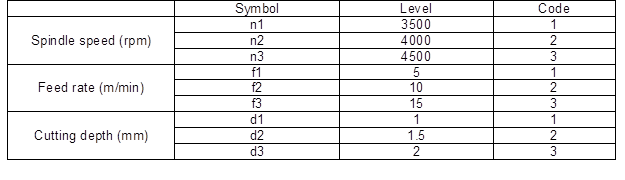Experimental Design

In this experiment, there were 3 parameter variables. Each parameter level was 3, and the number of normal experiments was 3*3= 81 (each group of experiments was repeated three times). To reduce the number of experiments and ensure adequate information, orthogonal experiments were used to design the experimental scheme (Xiao et al. 2016). In this study, 4 columns and 9 rows of L9 orthogonal tables were selected, and 9 parameter combinations were available. Therefore, the design experiment of the Lorthogonal array only needed 9*3 = 27 times. As shown in Table 2, a column array is empty for the experimental error, which does not affect orthogonality.

Table 2. LOrthogonal Experiment Table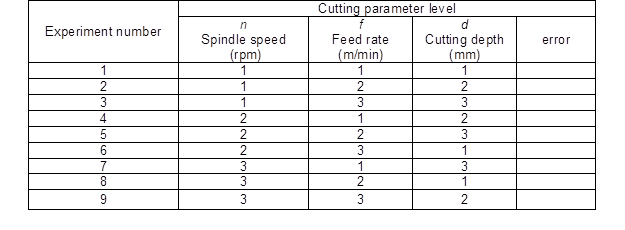RESULTS AND DISCUSSION

The orthogonal test results of the surface roughness are shown in Table 3. The range analysis, main effect analysis, range analysis, interaction analysis, variance analysis, and mathematical modeling analysis were conducted.

Table 3. Surface Roughness Test Results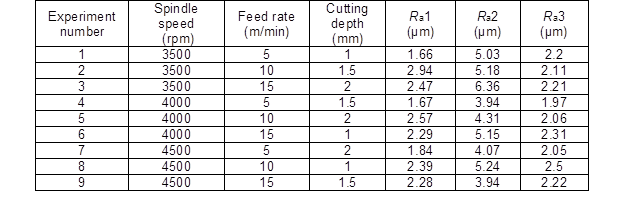Range Analysis

The effect of each cutting parameter on the surface roughness of bamboo can be separated at the different levels of the cutting parameters. The average surface roughness of experiment numbers 1-3, 4-6, and 7-9 were used as the average roughness of the spindle speed at levels 1, 2, and 3. Similarly, the average roughness of each level of other cutting parameters was calculated, as listed in Table 4. In the analysis table of average roughness range, the total average surface roughness of the tangential, cross, and radial sections of the bamboo was calculated.

Table 4. Range Analysis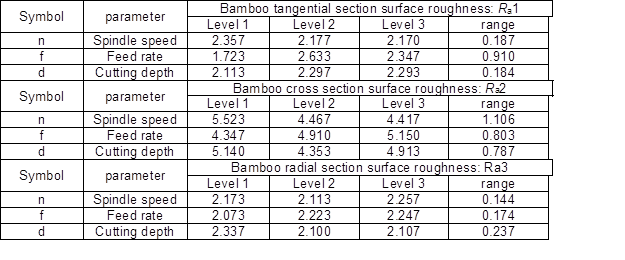Total average of Ra1: 2.234 μm, Standard deviation of Ra1:0.244μm;

Total average of Ra2: 4.802 μm, Standard deviation of Ra2:0.425μm;

Total average of Ra3: 2.181 μm, Standard deviation of Ra3:0.090μm.

According to the analysis results, the feed speed had the most significant effect on Ra1. Among the three factors, the effect of spindle speed on Ra1 was the second, and the cutting depth was the least. The effect of spindle speed on Ra2 was the most significant followed by the feed speed of Ra2, and then cutting depth. The cutting depth had the most significant effect on Ra3, followed by the feed speed, and the spindle speed was the least significant factor. Based on the growth characteristics of bamboo, the surface roughness of the string surface and diameter surface of bamboo was generally lower than that of the end surface. The total average surface roughness of Ra1 was 2.234 μm, for Ra2 it was 4.802 μm, and for Ra3 it was 2.181 μm. The standard deviation surface roughness of Ra1 was 0.244μm, for Ra2 it was 0.425 μm, and for Ra3 it was 0.090 μm.

Main Effect Analysis

The effect of surface roughness of bamboo is shown in Fig. 3. Generally, the better surface machining quality (Ra minimum) generally appears at the higher spindle speed, lower feed rate, and lower cutting depth. With the increase of spindle speed, the cutting amount per unit time increases, which improves the machining accuracy and surface quality. However, if the spindle speed is too high, the tool wear will be accelerated and the surface quality will be reduced. With the increase of feed rate, the machining amount per revolution of tool increases; that is to say, the amount of material cut from the workpiece by the tool per revolution becomes larger, which will reduce the surface quality, but the low feed rate will significantly affect the processing efficiency. Cutting depth and feed rate have similar effects on surface quality and machining efficiency. As shown in Fig. 3 (a), the feed speed highly affected Ra1. When the feed speed was increased from 5 m/min to 10 m/min, the surface roughness showed an upward trend, but when the feed speed was greater than 10 m/min, the surface roughness decreased slightly with the increase of the feed speed.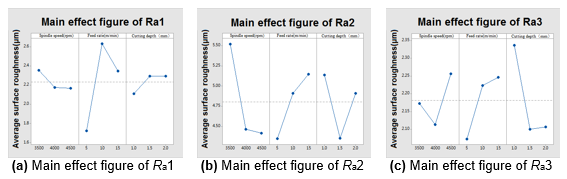Fig. 3. Main effect figure of average surface roughness Ra

As shown in Fig. 3 (a), the highest surface roughness occurred at a feed speed of 10 m/min, and the lowest surface roughness occurred when the feed speed was at 5 m/min, that is, at the best surface quality. With an increase in spindle speed, the surface roughness value decreased. The highest surface roughness occurred at a spindle speed of 3500 rpm, and the lowest surface roughness occurred at a spindle speed of 4500 rpm. As the cutting depth increased from 1.0 mm to 1.5 mm, the surface roughness increased rapidly. The highest surface roughness occurred at a cutting depth of 1.0 mm, and the lowest surface roughness was at a cutting depth of 1.5 mm, which was the best surface quality. When the cutting depth was increased from 1.5 mm to 2.0 mm, the surface roughness decreased slightly. These results suggested that the combination of the cutting parameters for the best surface roughness of Ra1 was the level-3 spindle speed, level-1 feed speed, and level-1 cutting depth.

As shown in Fig. 3 (b), the spindle speed had a strong influence on the surface roughness of the bamboo cross-section. The surface roughness decreased as the spindle speed increased from 3500 rpm to 4000 rpm. When the spindle speed was greater than 4000 rpm, the surface roughness increased rapidly with the increase of the spindle speed. The highest surface roughness occurred at a spindle speed of 4500 rpm, and the lowest surface roughness occurred at a spindle speed of 4000 rpm, which was the best surface quality. With an increase in feed speed, the surface roughness value showed an upward trend. The highest surface roughness occurred at a feed speed of 15 m/min, and the lowest surface roughness occurred at a feed speed of 5 m/min. When the cutting depth increased from 1.0 mm to 1.5 mm, the surface roughness decreased rapidly, and when the cutting depth increased from 1.5 mm to 2.0 mm, the surface roughness increased slightly. The highest surface roughness occurred when the cutting depth was 1 mm, and the lowest surface roughness occurred when the cutting depth was 1.5 mm, which implies the best surface quality. From the above analysis, it was concluded that the combination of the cutting parameters for the best surface roughness of Ra2 was the level-3 spindle speed, the level-1 feed speed, and the level-1 cutting depth.

As shown in Fig. 3 (c), the cutting depth strongly affected Ra3. When the cutting depth increased from 1.0 mm to 1.5 mm, the surface roughness decreased rapidly, and when the cutting depth increased from 1.5 mm to 2.0 mm, the surface roughness increased slightly. The highest surface roughness occurred when the cutting depth was 1 mm, and the lowest surface roughness occurred when the cutting depth was 1.5 mm, which was the best surface quality. With the increase in feed speed, the surface roughness value showed an upward trend. The highest surface roughness occurred at a feed speed of 15 m/min, and the lowest surface roughness occurred at a feed speed of 5 m/min. The surface roughness decreased when the spindle speed increased from 3500 rpm to 4000 rpm. When the spindle speed was greater than 4000 rpm, the surface roughness increased rapidly with the increase in the spindle speed. The highest surface roughness occurred at a spindle speed of 4500 rpm, and the lowest surface roughness at a spindle speed of 4000 rpm, which was the best surface quality. According to the above analysis, it was concluded that the cutting parameters of level-2 spindle speed, level-1 feed speed, and level-2 cutting depth are the best combination of the surface roughness of Ra3.

Variance Analysis

The effect of spindle speed, feed speed and cutting depth on the bamboo surface roughness was analyzed using variance analysis to determine the significance level of each variable. The analysis of variance of the test results of the surface roughness of bamboo (Ra1, Ra2, and Ra3) is shown in Table 6.

Through the evaluation of Ra1, it was found that the variable that had the greatest effect on the Ra1 value was the feed speed with a contribution rate of 45.39%. Other variables affecting Ra1 were spindle speed and cutting depth, with contribution rates of 2.34% and 2.31%, respectively, and with errors of 49.965% (Table 6). The feeding speed (factor B) was the factor that mostly affects Ra1. The influence of spindle speed (factor A) and cutting depth (factor C) on Ra1 was not significant in the given range.

Table 6. Surface Roughness Variance Analysis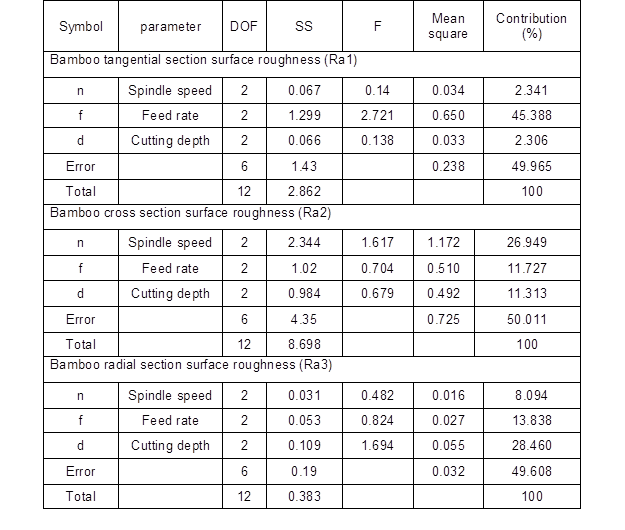Through the evaluation of Ra2, it was found that the variable that had the greatest influence on the value of Ra2 was the spindle speed with the contribution rate of 26.95%. The contribution rates of the feed speed and the cutting depth of Ra2 were 11.73% and 11.31%, respectively, and with an error of 50.01% (Table 6). The spindle speed (factor A) is the factor that mostly affects Ra2. The effects of the feed speed (factor B) and the cutting depth (factor C) on the Ra2 were significant and comparable in the given range.

Through the evaluation of the surface roughness Ra3, it was determined that the cutting depth had a contribution rate of 28.46% and had the greatest effect on the value of Ra3. It was found that the contribution rates of spindle speed and feed speed of Ra3 are 8.09% and 13.84%, respectively, with an error of 49.61% (Table 6). The cutting depth (factor C) is the factor that most affected Ra3. The feeding speed (factor B) had a significant effect on Ra3 in a given range. The effect of spindle speed (factor A) on Ra3 was not significant within the given range.

Interaction Analysis

The results of the interactive effect on the surface roughness of the tangential, cross, and radial sections of bamboo are shown in Table 5. The interaction effect diagram is shown in Fig. 4. Each interaction effect diagram shows the interaction diagram of the combination of two cutting parameters.

Table 5. Surface Roughness Interaction Effect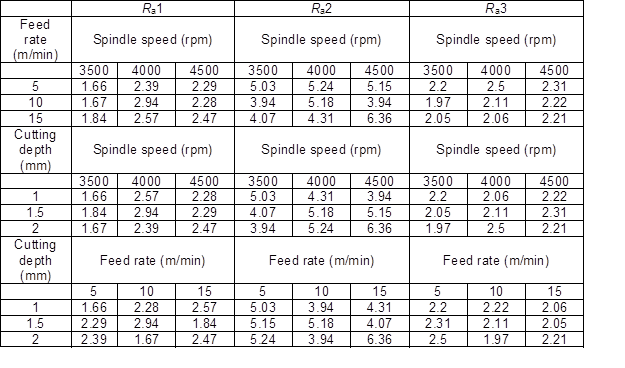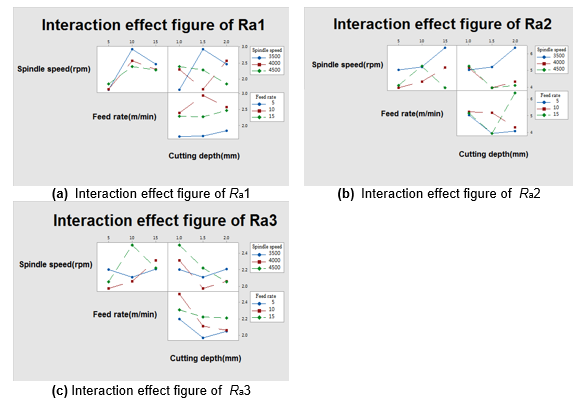Fig. 4. Interaction effect figure of average surface roughness Ra

In Fig. 4 (a), the top row of the two pictures from left to right shows the three spindle speeds (3500 rpm, 4000 rpm, 4500 rpm), the average surface roughness, the feed rate, and cutting depth of interaction diagram. The second line graph shows the three feed speed (5 m/min, 10 m/min, 15 m/min) from the average Ra1 and the cutting depth of the interaction diagram. All three interaction diagrams show non-parallel lines, indicating an interaction between cutting parameters. Similarly, all interaction diagrams in Fig. 4 (b) and (c) show non-parallel lines, indicating that there is also an interaction between cutting parameters.

Mathematical Modeling and Analysis

The response surface method was used to model and analyze the experiment, and the mathematical model of the bamboo milling process was established by the same method. MINITAB 17.0 software was used for calculations. To study the effect of cutting parameters on surface roughness, the regression equations of Ra1, Ra2, and Ra3 were derived according to the test data, as follows,

y=α1+α2*x1+α3*x2+α4*x3 (1)

where y represents the surface roughness, x1x2, and x3 refer to the cutting parameters. The term refers to the regression coefficient. The first-order model can be expressed as a function of the cooling conditions associated with the cutting parameters (spindle speed, feed speed, and cutting depth) and surface roughness Ra. The relationship between surface roughness Ra and milling parameters is shown in Eq. 2.

y=α1+α2*n+α3*f+α4*d (2)

The mathematical model between surface roughness Ra and milling parameters was established by replacing the numerical value in Eq. 2 with the experimental results, and the surface roughness Ra model is obtained below.

Ra1 =2.09-0.000187*n+0.0623 *f+0.18*d (3)

Ra2 =8.77-0.001107*n+0.0803* f-0.227*d (4)

Ra3 =2.019+0.000083*n+0.01733*f-0.23*d (5)

The measurement coefficient R2 is a measure of the change in the mean value of the model’s interpretation. For Ra1, Ra2, and Ra3, the Rvalues were 45.60%, 55.43%, and 66.59%, respectively.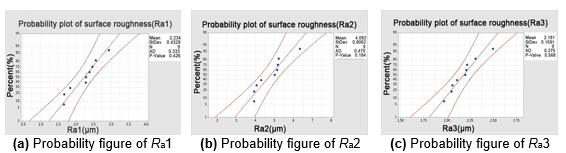Fig. 5. Probability figure of surface roughness

Figure 5 shows the probability graph of surface roughness. In Fig. 5(a), the probability line explains that the surface roughness value of almost 70% of the bamboo’s tangential section was lower than 2.5 μm, the average surface roughness of the bamboo’s tangential section was 2.234 μm, and with a standard deviation of 0.4329. Because the data points were roughly along a straight line, the P-value was greater than 0.05, and the AD statistical value was low. Therefore, it can be inferred that the data were from a normal distribution. Figures 5(b) and 5(c) have similar rules with Fig. 5(a), and Ra1, Ra2, and Ra3 data obtained from a normal distribution.

Because the data of Ra1, Ra2, and Ra3 were all from the normal distribution, the response surface of the second-order model can be fitted as Eq. 6,

y=α1+α2*x1+α3*x2+α4*x3+α5*x12+α6*x22+α7*x32+α8*x1*x2+α9*x1*x3+α10*x2*x3 (6)

where y represents the surface roughness, x1x2, and x3 refer to the cutting parameters, and the regression coefficient refers to the parameter. The second-order model can be expressed as a function of the cooling conditions associated with the cutting parameters (spindle speed, feed speed, and cutting depth) and surface roughness Ra. Since f*d cannot be estimated, it is deleted. The relationship between surface roughness Ra and cutting parameters is shown in Eq. 7.

y=α1+α2*n+α3*f+α4*d+α5*n2+α6*f2+α7*d2+α8*n*f+α9*n*d (7)

The mathematical model between the surface roughness, Ra and the cutting parameters is established by replacing the numerical value in Eq. 7 with the experimental results, as shown below.

Ra1 = 5.16 – 0.003293*n+ 0.9297*f – 0.6467*d + 3.4667*10-7*n2– 0.02753*f-0.7467*d2-7.467*10-5 *n*f + 0.00072 *n*d; (8)

Ra2 = 30.64 – 0.01344*n+ 0.617*– 0.36* d + 2.013*10-6 *n2+ 0.001133* f2 + 1.9467d2 – 0.000149*n*f – 0.00152*n*d; (9)

Ra3 = 7.83 – 0.002763*n + 0.02767*f – 0.5867 *d + 4.0667*10-7*n2– 0.001133 *f2+ 0.4933*d2+ 1.333*10-6*n*f– 0.00028*n*d (10)

Because the degree of freedom of the error column is 0, the values of feed speed and cutting depth cannot be calculated. One or more interaction terms will be deleted and the model will be fitted again. According to Eq. 8, the absolute value of the interaction term coefficient, n*f is 7.467*10−5, which is less than the absolute value of the interaction term coefficient, n*d which is 0.00072. Therefore, the n*f term is deleted and the Ra1 model is fitted again. According to Eq. 9, the absolute value of the interaction term coefficient, n*f is 0.000149, which is less than the absolute value of the interaction term coefficient, n*d which is 0.00152. Therefore, the n*f term is deleted and the Ra2 model is fitted again. According to Eq. 10, the absolute value of the interaction term coefficient, n*f is 1.333*10−6, which is less than the absolute value of the interaction term coefficient, n*d that is 0.00028. Thus, the n*is deleted and the Ra3 model is fitted again. The modified model is as shown below.

Ra1= 6.653 – 0.00348*n + 0.584333*– 0.0867*d + 3.467*10-7*n2– 0.02567*f– 0.3733*d2+ 0.0003467*n*d; (11)

Ra2= 33.6267 – 0.0138133*n – 0.07367*f + 0.76*d + 2.0133*10-6*n2+ 0.004867*f2 + 2.6933*d2 – 0.002267*n*d; (12)

Ra3 = 7.803 – 0.00276*n + 0.03383*f – 0.5967*d + 4.067*10-7*n2– 0.001167*f2 + 0.4867*d2 – 0.000273*n*d (13)

The model of surface roughness parameter Ra is shown in Eqs. 11, 12, and 13, and its measurement coefficients of determination are R= 96.5%, 96%, and 100% respectively, and are close to uniform. This means that the revised model can fit the actual data better and can explain the extent to which the model is reliable.

The surface roughness and contour diagram are shown in Figs. 6, 7, and 8, clearly indicating the relationship between each cutting parameter.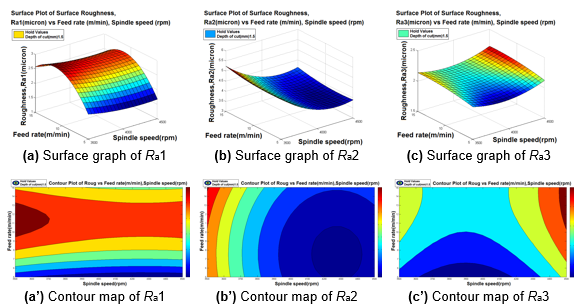Fig. 6. Influence of spindle speed and feed speed on surface roughness (d=1.5 mm)

Figure 6 shows the effect of the spindle speed (n) and feed speed (f) on the surface roughness of bamboo with the cutting depth (d) at a medium level (d = 1.5 mm). High spindle speed and low feed speed produces an optimal Ra1, while high spindle speed and low feed speed produces an optimal Ra2. High Ra2 occurred at a low spindle speed and at a high feed speed. The medium spindle speed and low feed speed produces an optimal Ra3 and occurred at a high spindle speed and a high feed speed. The analysis results are the same as above.

Figure 7 shows the effect of spindle speed (n) and cutting depth (d) on the surface roughness of bamboo with the feed speed (f) at an intermediate level (f = 10 m/min). High spindle speed and low feed speed produces an optimal Ra1. According to Fig. 7(b) and (b’), high spindle speed and high feeding speed produces an optimal Ra2, and a high Ra2 occurred at a low spindle speed and a high feeding speed. It was deduced from Fig. 7 (c) and (c’) that the medium spindle speed and high feeding speed produces an optimal Ra3, and a high Ra3 occurred at a high spindle speed and a low feeding speed. The analysis results are the same as above.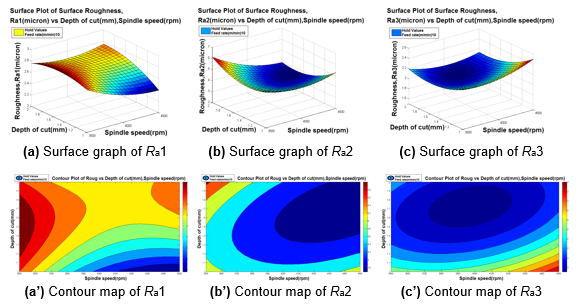Fig. 7. Influence of spindle speed and cutting depth on surface roughness (f=10 m/min)